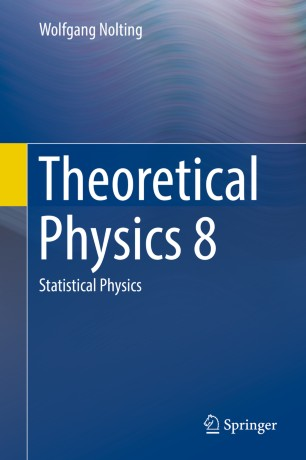# Theoretical Physics 8

## Statistical Physics

• Wolfgang NoltingTextbook

1. Front Matter
Pages i-xiv
2. Wolfgang Nolting
Pages 1-93
3. Wolfgang Nolting
Pages 95-161
4. Wolfgang Nolting
Pages 163-268
5. Wolfgang Nolting
Pages 269-404
6. Back Matter
Pages 405-638

### Introduction

This textbook offers a clear and comprehensive introduction to statistical physics, one of the core components of advanced undergraduate physics courses. It follows on naturally from the previous volumes in this series, using methods of probability theory and statistics to solve physical problems. The first part of the book gives a detailed overview on classical statistical physics and introduces all mathematical tools needed. The second part of the book covers topics related to quantized states, gives a thorough introduction to quantum statistics, followed by a concise treatment of quantum gases.

Ideally suited to undergraduate students with some grounding in quantum mechanics, the book is enhanced throughout with learning features such as boxed inserts and chapter summaries, with key mathematical derivations highlighted to aid understanding. The text is supported by numerous worked examples and end of chapter problem sets.

Translated from the renowned and highly successful German editions, the eight volumes of this series cover the complete core curriculum of theoretical physics at undergraduate level. Each volume is self-contained and provides all the material necessary for the individual course topic. Numerous problems with detailed solutions support a deeper understanding. Nolting is famous for his refined didactical style and has been referred to as the "German Feynman" in reviews.

### Keywords

Textbook Nolting Textbook Theoretical Physics Textbook Statistical Physics Textbook Quantum Gas Textbook Phase Transition Introduction Statistical Physics

#### Authors and affiliations

• Wolfgang Nolting
• 1
1. 1.Institute of PhysicsHumboldt-University at BerlinGermany